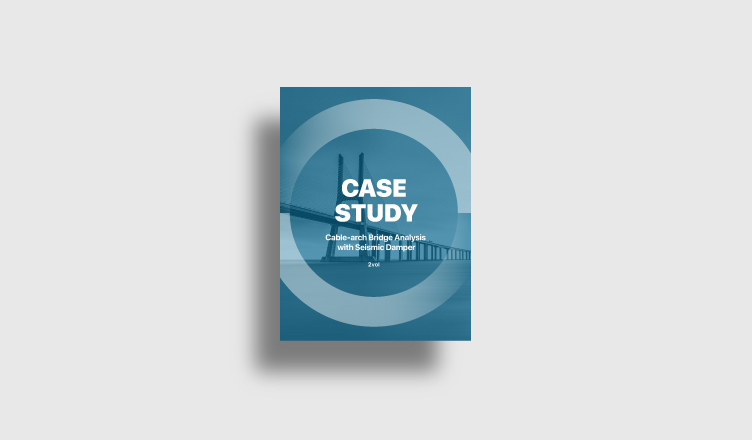BLOG BRIDGE INSIGHT

With the advent of the IT era in the 21st century, information that was previously unavailable in the engineering market has become possible. Engineering simulation using a computer program is one of them, and it is currently used in most engineering industries.

In order to perform a correct structural analysis simulation using such a computer analysis program, it is necessary to compare and verify the contents of engineering theory and the results calculated by the program. It is an essential process in industries such as construction and transportation, which are directly related to human safety.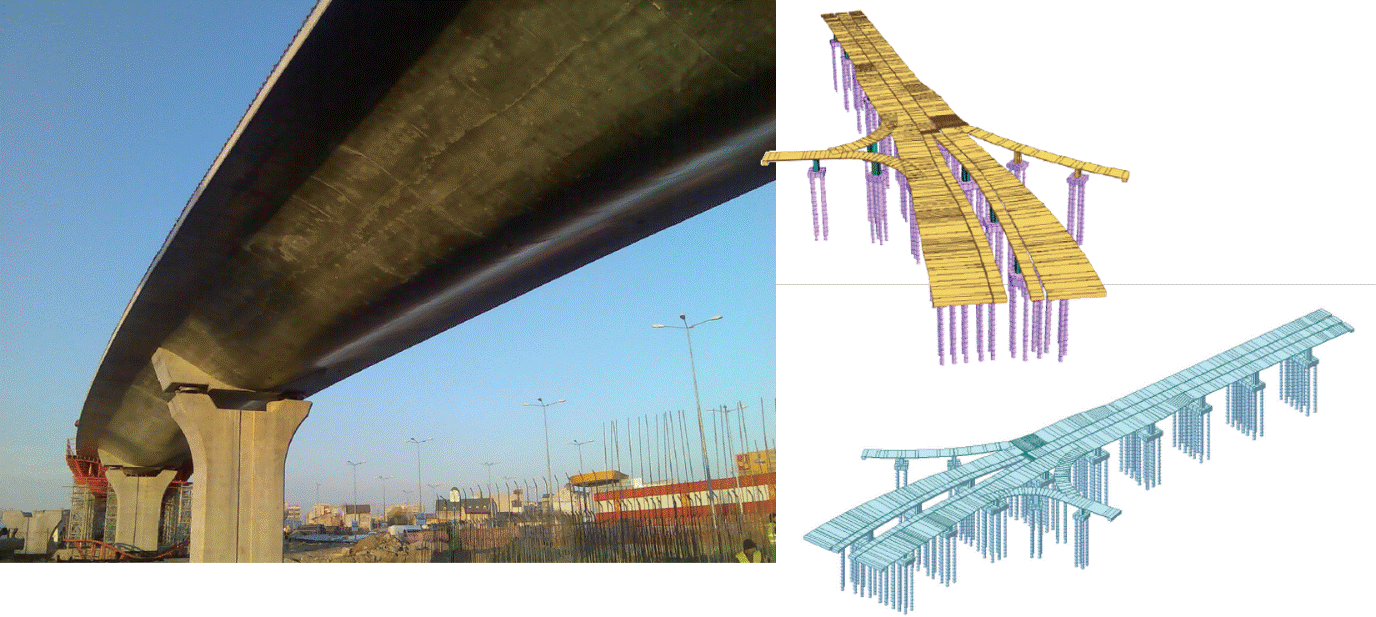In this post, we will compare the theoretical contents to the analysis results in a structural analysis program about the prestressed concrete equilibrium equation and the prestressed tendon loss.

PSC bridge is a bridge type that secures the stability of the structure by introducing artificial deformation and stress using tendon to the concrete member. The stress introduction by tendon depends on the tendon shape and tension. The shape of the tendon is defined as the distance from the neutral axis of the member. If the neutral axis changes for each construction stage, such as the composite section in the construction stage, this should be appropriately reflected.

Tension can be divided into immediate losses, such as friction or anchorage losses, and time-dependent losses that occur as the construction stage progresses, such as elasticity change or relaxation. Such tendon losses must be properly reflected in the analysis.

## 1. Verification of Equivalent Tendon Force

Assuming that there is no Tendon Losses, the equivalent tendon force is calculated as follows.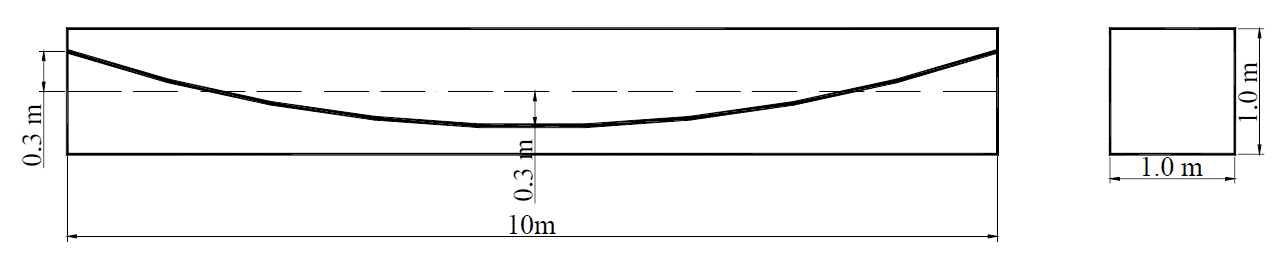Case. Simple beam model with the tendon placed in a curve.

Material and section properties are following:

 Modulus of Elasticity (Conc), Ec 10,000 Modulus of Elasticity (Steel) Es 150,000 Area of Steel Ast 0.01 Yield Stress Fy 100,000 Diameter of Duct dduct 0.04 m Tensile Stress fso 80,000 Eccentricity ei 0.3 m Span length L 10 m

### → Theoretical approach method and calculation results

#### ii. Here, the arrangement of the tendons in each section is assumed to be linear.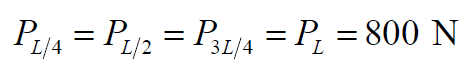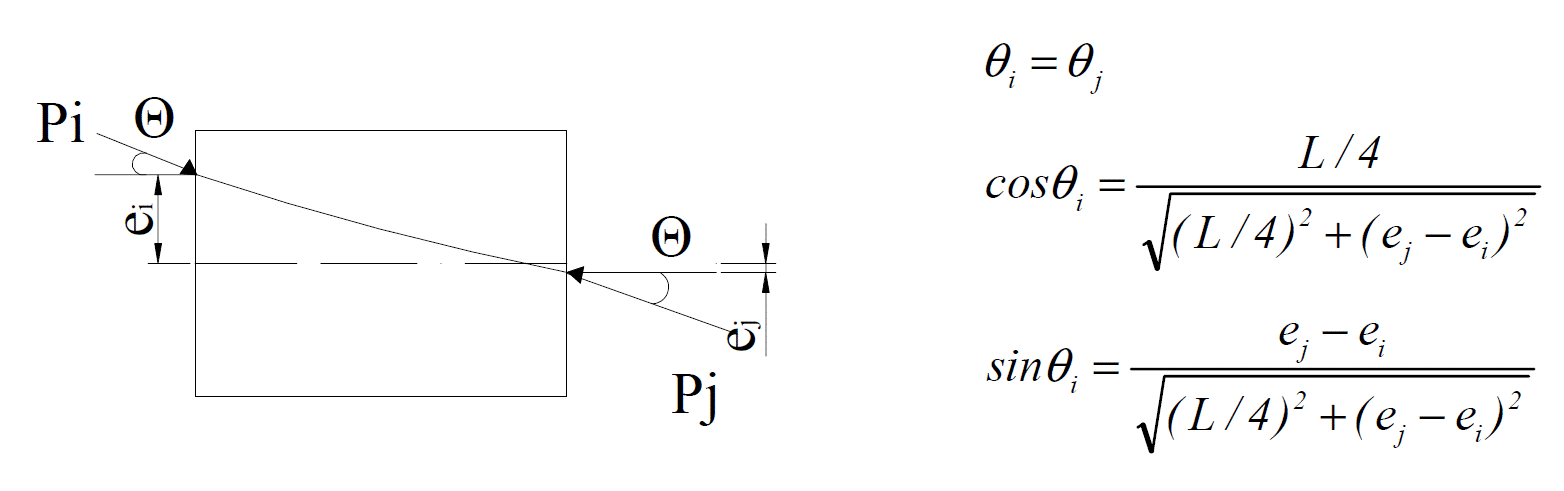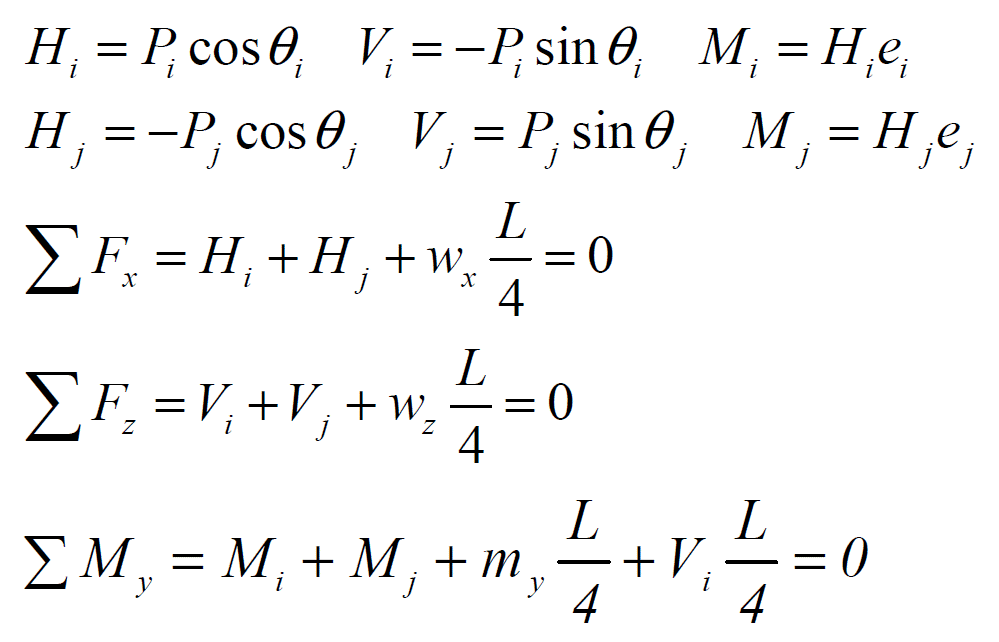### iii. The neutral axis moves because the area of the tension member and the duct is calculated as a transformed cross section. Where, each position is described as e0=i, e1=1/4, e2=2/4.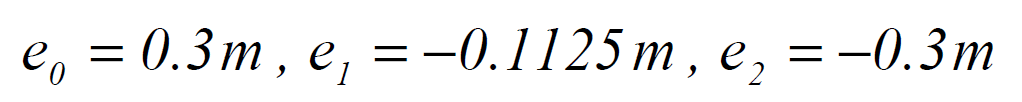After the change of the neutral axis by the effective section,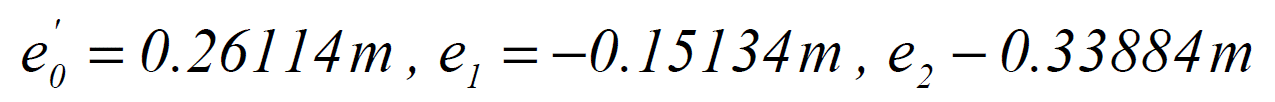#### iv. Where Pi=Pj and ei=ej,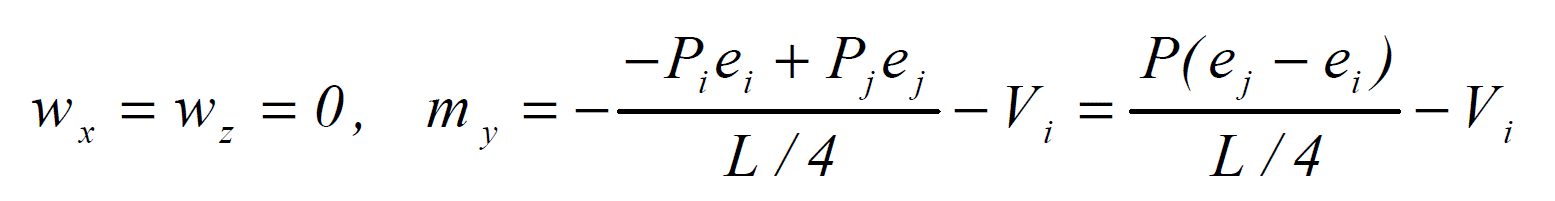Therefore, the equivalent tendon force acting on each section is shown in the below figure.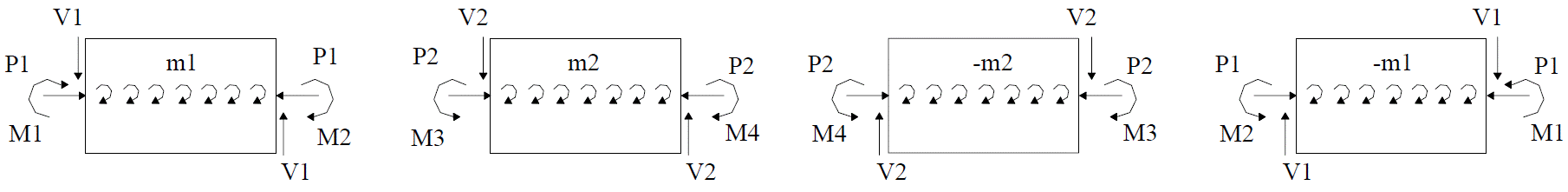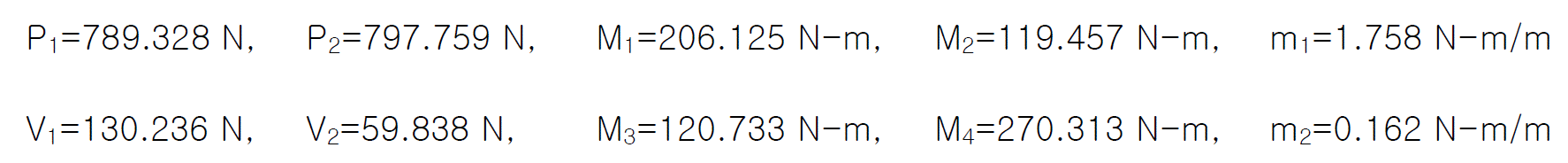### → Modelling and structural analysis result by midas Civil

#### i. Input Model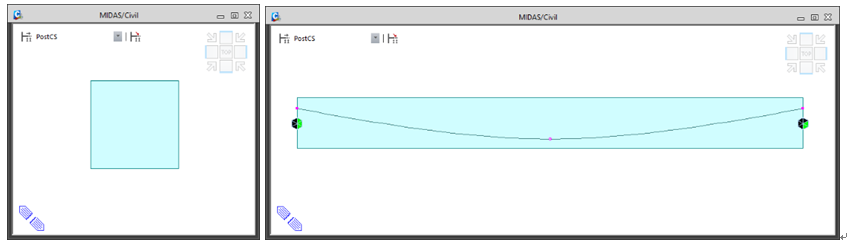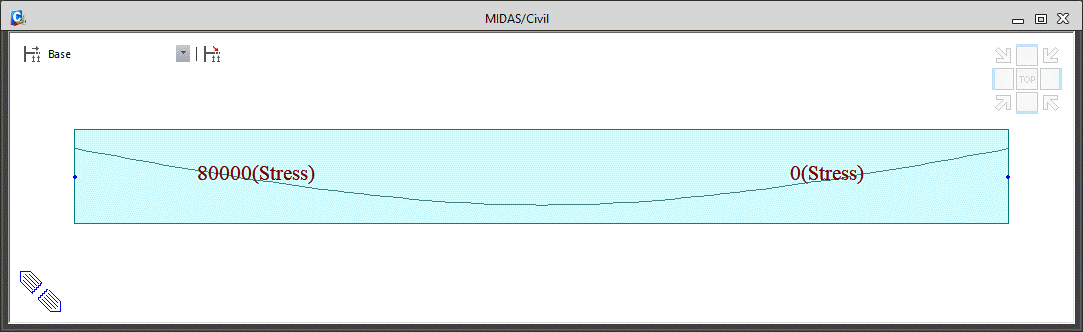#### iii. Analysis Results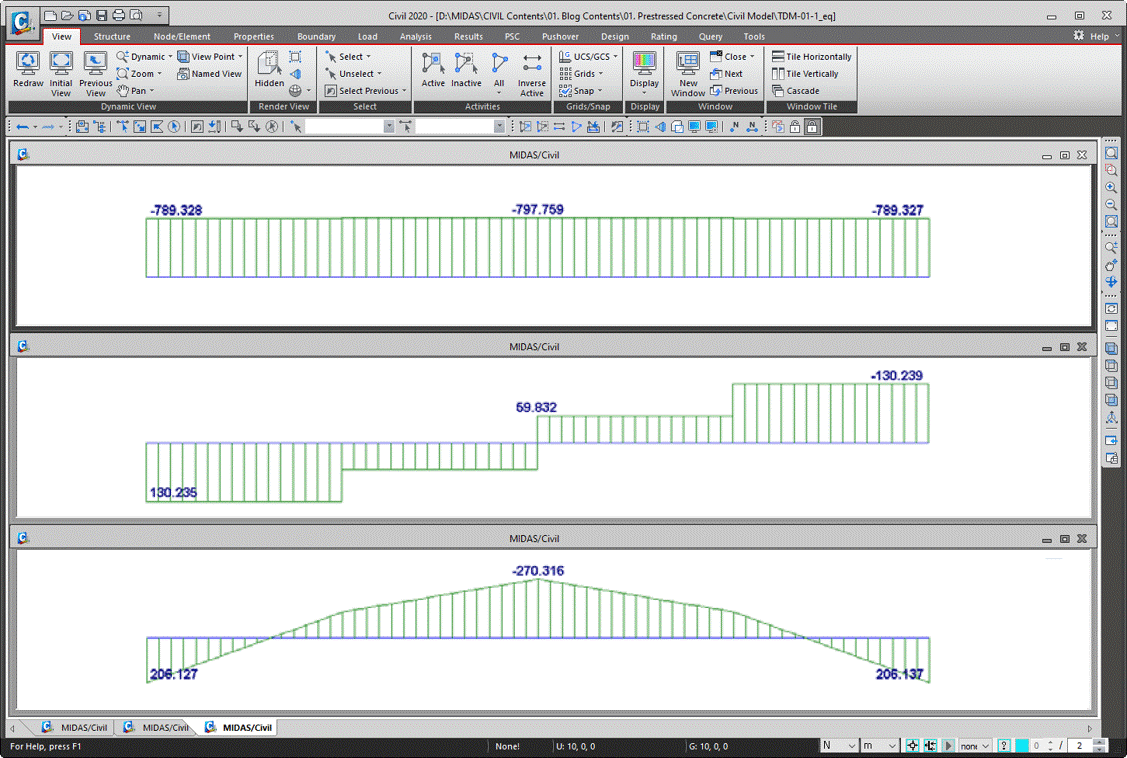### → Comparison of theoretical results and analysis results using midas Civil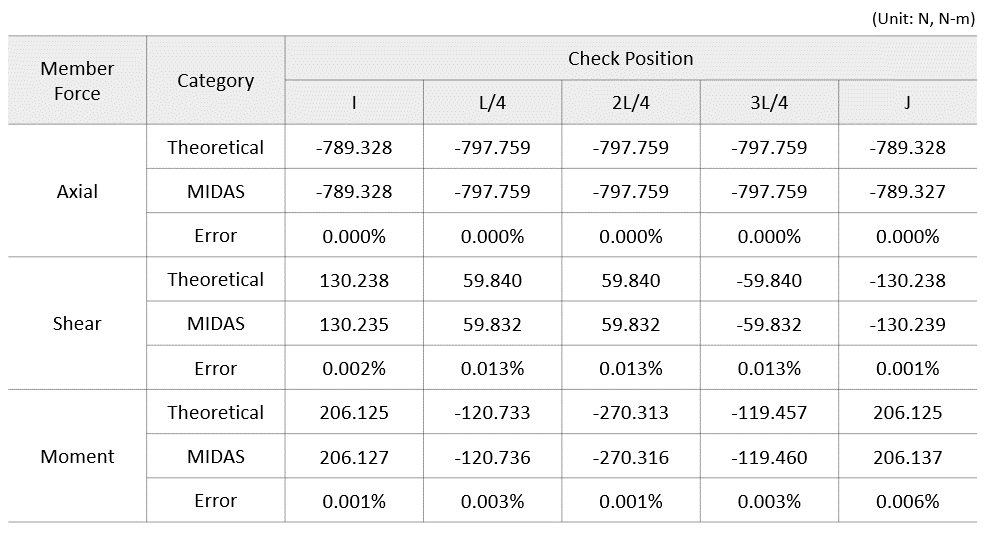## 2. Tendon Losses due to Friction, Anchorage Slip, and Relaxation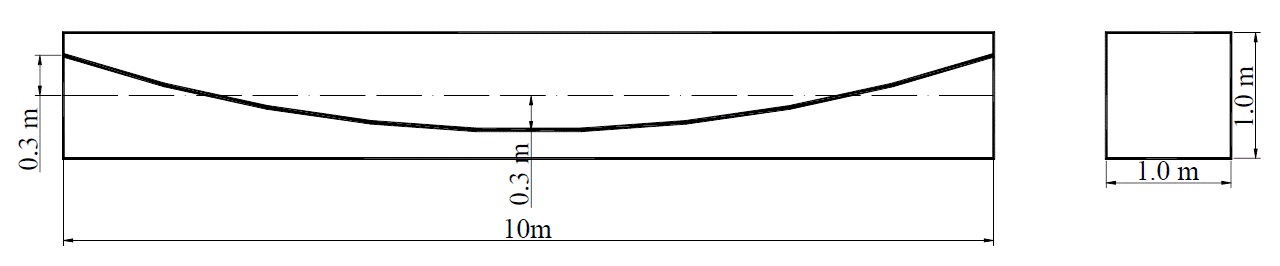Case. Simple beam model with the tendon placed in a curve

Material and section properties are following:

 Modulus of Elasticity (Conc) Ec 10,000 Anchorage slip Lslip 0.05 m Modulus of Elasticity (Steel) Es 150,000 Relaxation coefficient Crelax 45 Area of Steel Ast 0.01 Curvature friction factor μ 0.3 Yield Stress Fy 100,000 Wobble friction factor k 0.0066/m Diameter of Duct dduct 0.04 m t1 100 day Tensile Stress fso 80,000 Eccentricity ei 0.3 m ej 0.3 m Span length L 10 m

### →Theoretical approach method and calculation results

#### i) Loss due to friction

The tension at each L/4 point is as follows.

#### ii) Loss due to anchorage slip

Let the length of the tendon subject to reverse friction be set, calculate the tendon loss due to settlement activity based on where this length is in any of the four sections.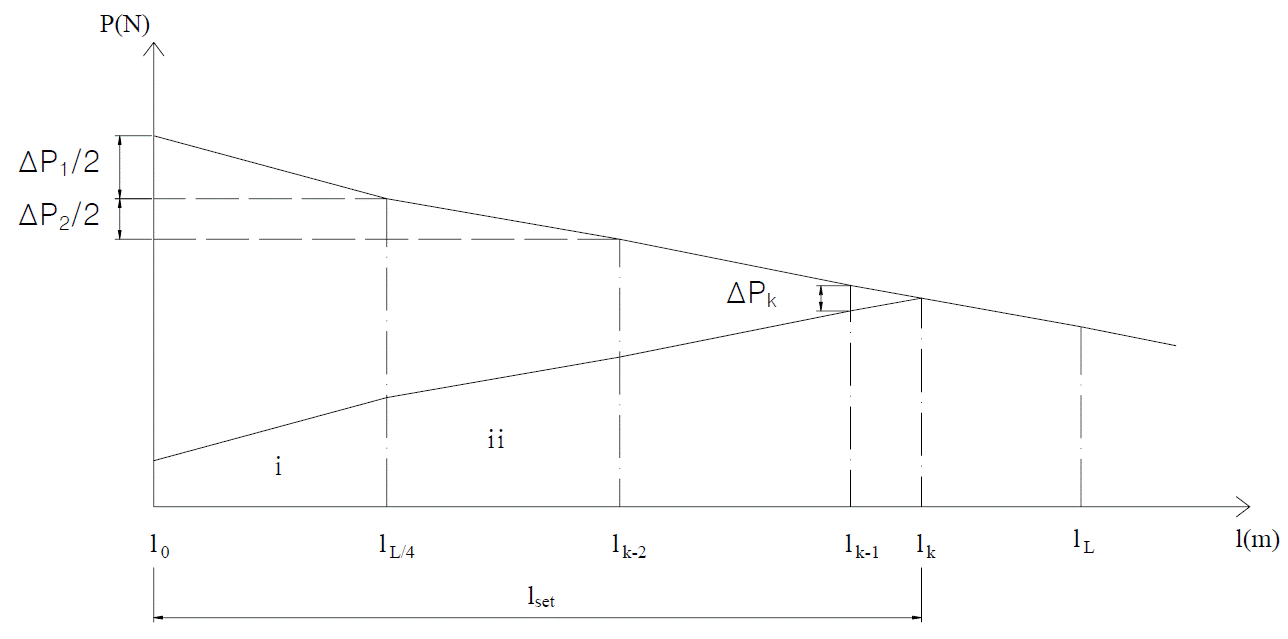Tendon loss graph by anchorage slip

(a) Section I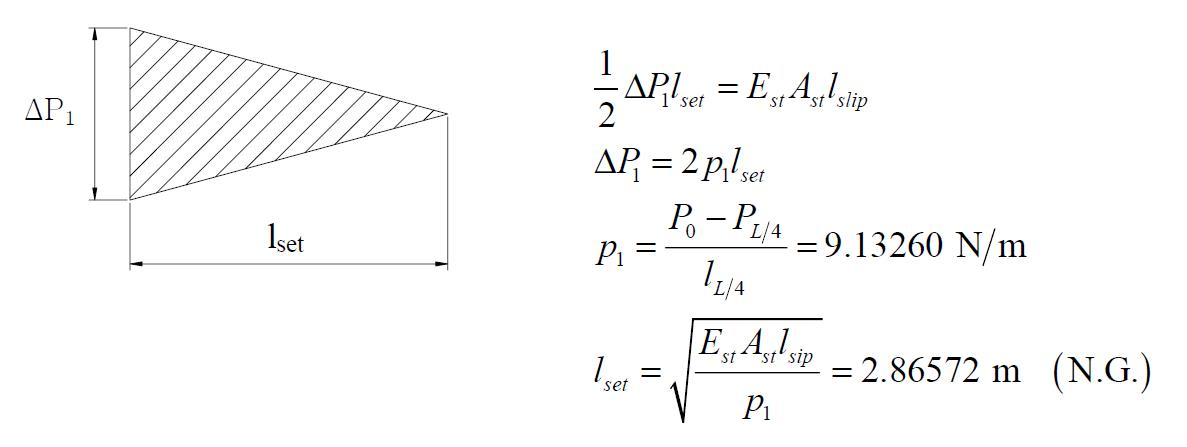(b) Section ii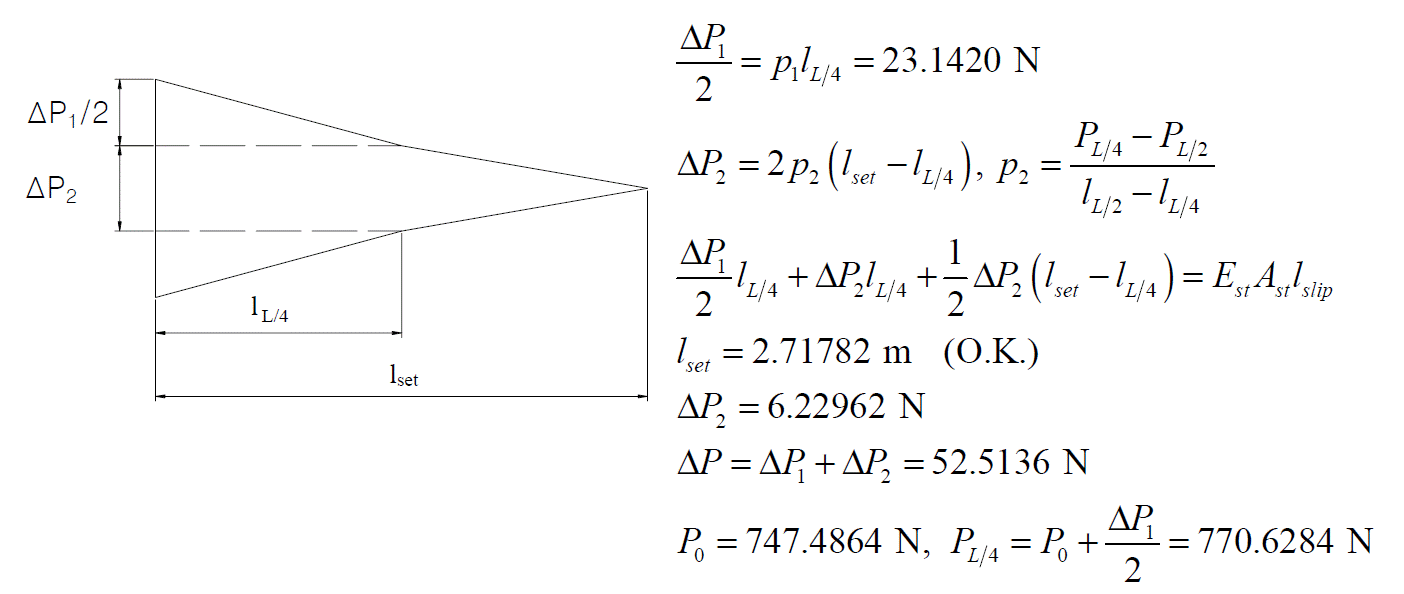The rest remains, PL/2, P3L/4, PL unchanged.

iii) Loss due to relaxation

Loss due to relaxation is calculated as follows.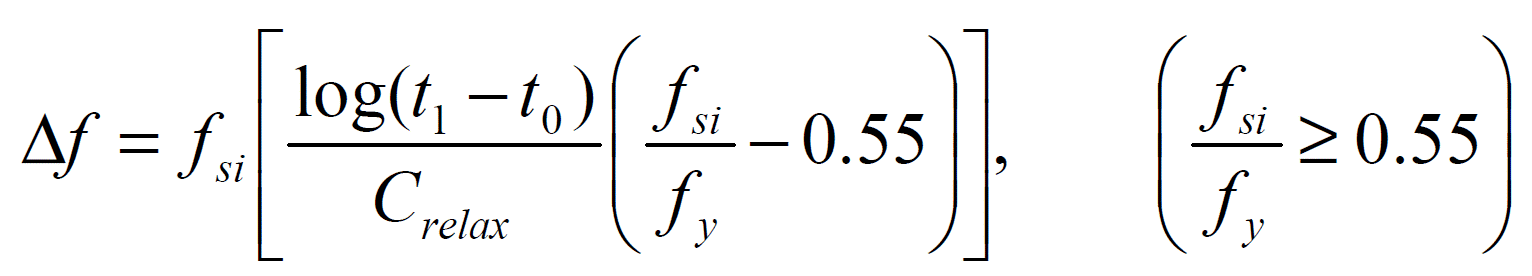Here, fsi is the stress before loss due to relaxation occurs, and t is the elapsed time (hr). Crelax is relaxation coefficient (45 in this example), and fy (100,000 N/m2) is yield stress.

Therefore, the tension force considering the loss due to relaxation at each point is as follows.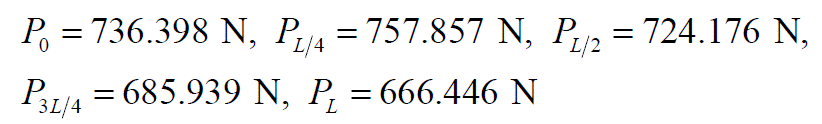### → Modelling and structural analysis result by midas Civil

#### i) Input Model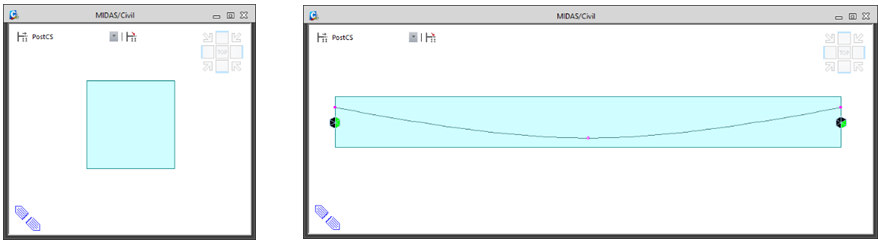#### iii) Analysis Results

(a) Loss due to friction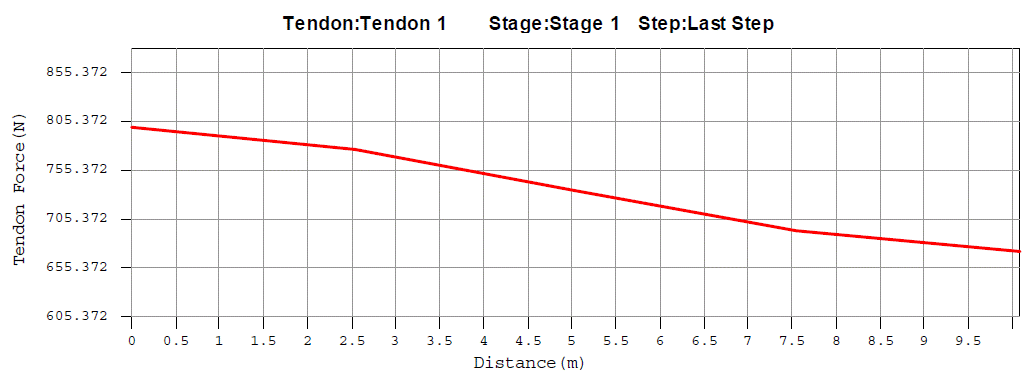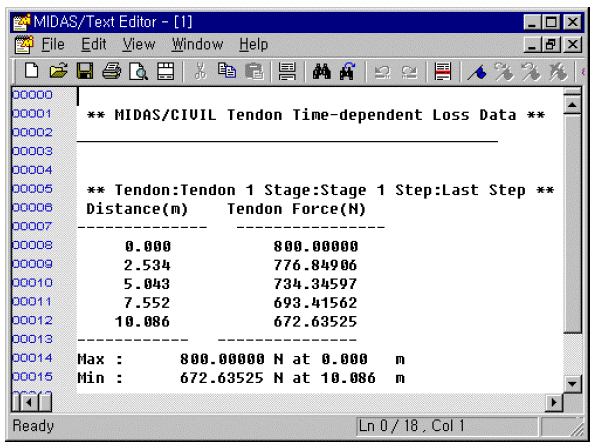(b) Loss due to anchorage slip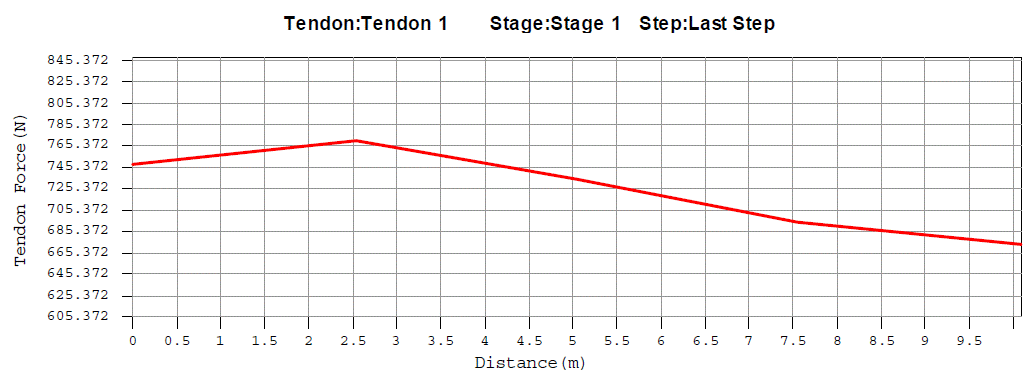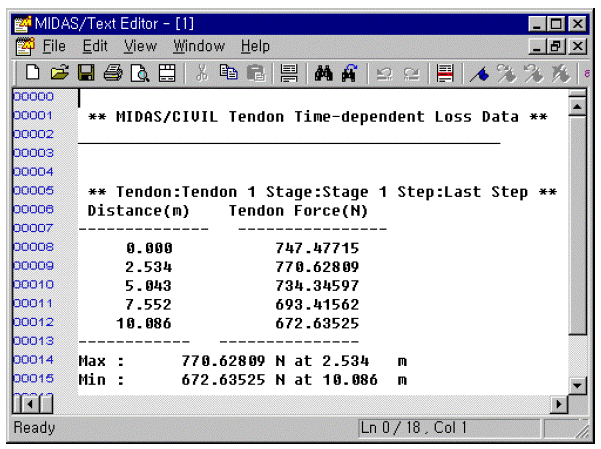(c) Loss due to relaxation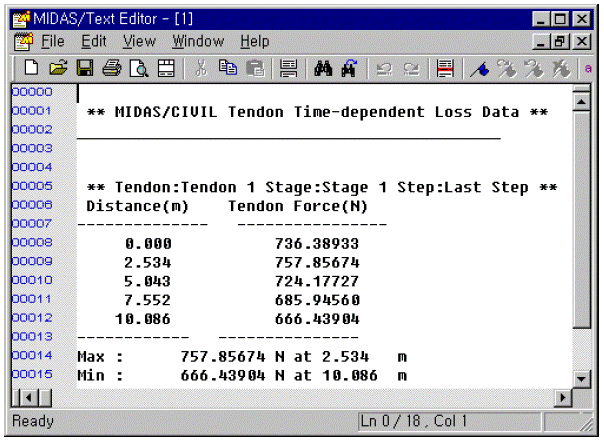### → Comparison of theoretical results and analysis results using midas Civil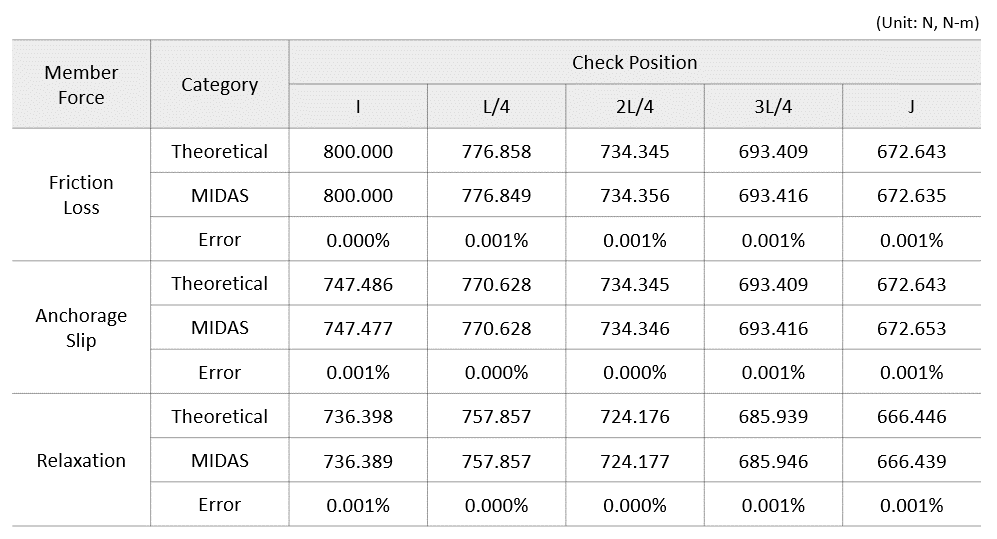TaeGook Kim | Technical Engineer | MIDASIT

- Civil engineer within MIDASIT Technical Planning Team

- Over 3 years of experience in technical and marketing for MIDAS Programs